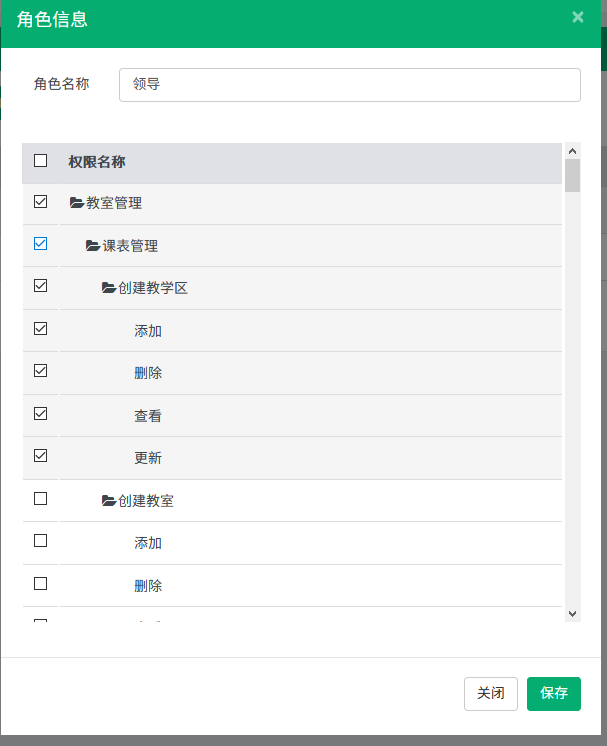``````function getRoleList() {

\$("input[name='btSelectItem']").each(function (d) {
if(\$(this).prop("checked")) {
console.log(\$(this).val());
if(\$(this).val()!="on") {
list2.push(\$(this).val());
console.log(list2.length);
}
}
});
}
function unSaveClose() {
var list = new Array();
\$("input[name='btSelectItem']").each(function (d) {
if(\$(this).prop("checked")){
if(\$(this).val()!="on"){
list.push(\$(this).val());

}
}
});
if(list.length!=list2.length)
//  if(list.length!=0)
{
btn: ['关闭',"取消"] //按钮
``````

2个回答10 个月之前 回复10 个月之前 回复weixin_40852944 这2个方法是怎么调用的
10 个月之前 回复

1. 数据模型, 负责记录/计算当前已经被勾选的数据
2. UI展示, 根据数据模型刷新UI视图
3. 整合, 联动数据和UI